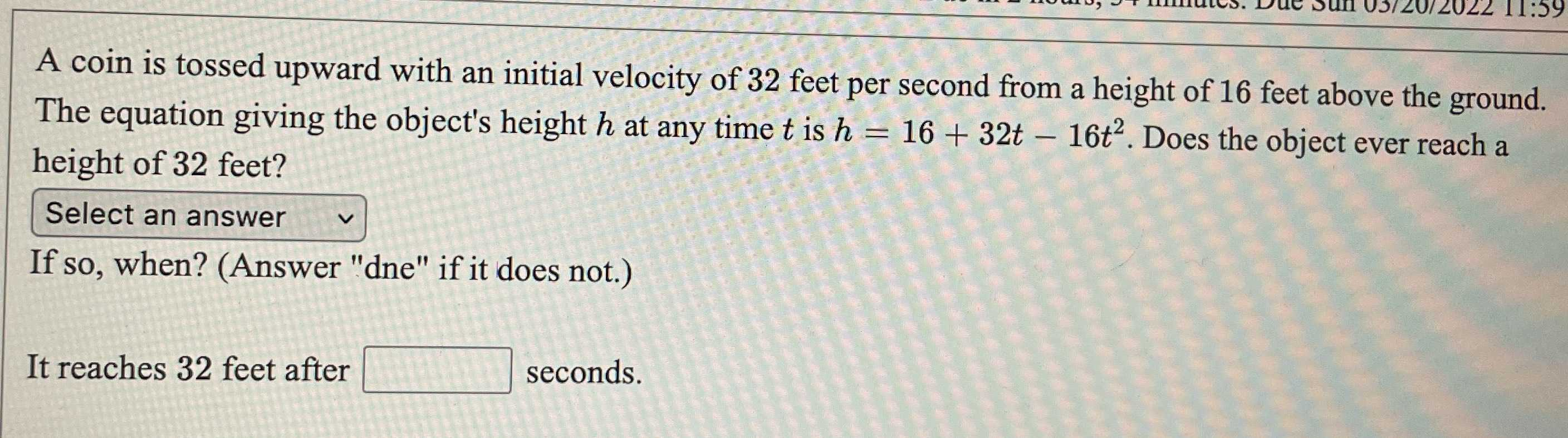### ¿Todavía tienes preguntas de matemáticas?

Pregunte a nuestros tutores expertos
Algebra
PreguntaA coin is tossed upward with an initial velocity of $$32$$ feet per second from a height of $$16$$ feet above the ground. The equation giving the object's height $$h$$ at any time $$t$$ is $$h = 16 + 32 t - 16 t ^ { 2 }$$ . Does the object ever reach a height of $$32$$ feet?